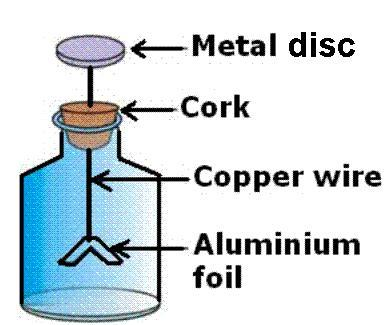## Monday, December 23, 2019

### Unizor - Physics4Teens - Electromagnetism - Carrier of Electricity

Notes to a video lecture on http://www.unizor.com

Carrier of Electricity

Electricity is a form of energy.
Mechanical energy is carried by moving objects. Thermal energy is carried by oscillating molecules. Electricity, as a form of energy, must have its carrier too.

As we know, any object consists of molecules that retain the object's properties. There are thousands of different molecules, corresponding to thousands of different in their characteristics objects.

Molecules are comprised from atoms, which have completely different characteristics. There are just a little more than a hundred different atoms, from their compositions all molecules are built.

Each atom, according to a classical model that we will use when talking about electricity, consists of a nucleus, where certain number of protons and neutrons are bundled together, and electrons that rotate on different orbits around a nucleus.

What force keeps electrons on their orbits?
Similarly to gravitational force that keeps planets on their orbits around a sun, there is a force between a nucleus and electrons circling on their orbits.
This force is called electric force. As in case of gravity, we can talk about electric field as a domain of space, where electric forces are acting.

Numerous experiments show that the attracting electric force exists between protons and electrons. Neutrons do not play any role in keeping electrons on their orbits. Further experiments showed that, while protons and electrons are attracted to each other, two protons repulse each other, and so are two electrons. That prompted the designation of terms positive and negative to describe the electric energy carried, correspondingly, by protons and electrons and the term electric charge to name and quantify this form of energy.

So, we say that protons carry positive electric charge, while electrons carry negative electric charge. Positive and negative electric charges attract and neutralize each other, while two positive or two negative charges repulse each other.

In a normal state atoms have equal number of protons and electrons, thus are electrically neutral, which implies that the amount of electric charge in one proton and one electron are equal, though opposite in "sign". Obviously, our designation of "positive" and "negative" is just for a convenience of description and ease of calculations.

Under some circumstances the number of electrons in an object might be greater or less than the number of protons, which causes the entire object to be negatively (when there is an excess of electrons) or positively (when there is a deficiency of electrons) charged.

In case of excess of electrons, some electrons are no longer attached to a nucleus, but travel freely inside the object. Because of the excess of electrons, the entire object becomes negatively charged.
When there is a deficiency in number of electrons, certain spots on certain orbits around certain nuclei remain empty. The entire object becomes positively charged.
If a negatively charged object A comes to contact with a positively charged object B, excess electrons from A might migrate to B, thus diminishing negative charge of A by diminishing excess of electrons and diminishing positive charge of B by filling empty spaces on the orbits of those atoms that had deficiency of electrons.

Electric force is really strong. As an example, let us consider two small cubes of iron of 1 cm³ each positioned at the distance of 1 meter from each other.
There are 8.4·1022 atoms in each cube. Each atom contains 26 protons, 30 neutrons and 26 electrons.
If we magically strip all atoms in one cube of iron of all their electrons and transfer them to another cube, the one with deficiency of electrons will be positively charged and the one with excess electrons will be negatively charged. So, they will attract each other.
The total number of excess electrons in one cube, which is equal to the total number of deficient electrons in another one is
26·8.4·1022 ≅ 2.2·1024
The distance between them is 1 meter. According to Coulomb Law, that we will study later, the force of attraction between these two small cubes of iron will be, approximately
F = 1.11·1021 N (newtons)
THIS IS HUGE!
Just as a comparison, the force of gravity that keeps the planet Mars on its orbit around the Sun is equal to 1.6·1021 N, which is quite comparable.
It also shows how much stronger electrical forces are, compared to gravitational.

Fortunately, we never have to deal with such strong forces, all atoms that comprise an object are never stripped of all their electrons.

It's easy to see electricity in action.
Lightning is the flow of electrons from the negatively charged cloud to some object on the ground.
Getting off some synthetic clothes is accompanied by small visible in the dark and audible sparks, which are also the flows of electrons from an object with excess of electrons to an object with their deficiency.

An electroscope is a device that can demonstrate the excess or deficiency of electrons, that is whether an object is electrically charged.Initially, the electroscope is electrically neutral and aluminum foils are in vertical position, as there is no electrical force between them.
If electrically charged object touched the metal disc of an initially neutral electroscope, excess or deficiency of electrons in an object will be shared with metal disc and, further, with aluminum foils. This will negatively or positively charge both aluminum foils, and they will split apart because the same electrical charge (positive or negative) repulse objects (aluminum foils in this case).
With proper calibration this effect can be used to measure the amount of electricity by the angle of deviation of aluminum foils from vertical, as more electrons are in excess or deficiency - the stronger the repulsive force will be between the foils, and the greater angle of deviation of their position from the vertical will be.